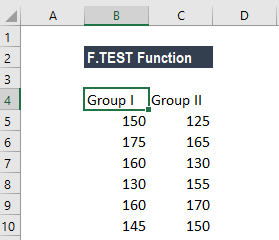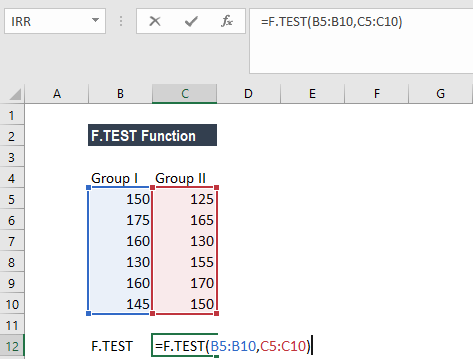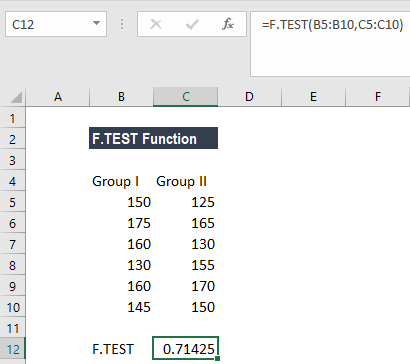# F.TEST Function

Returns the result of an F-test for two given arrays or ranges

## What is the F.TEST Probability Function?

The F.TEST function is categorized under Excel Statistical functions. It will return the result of an F-test for two given arrays or ranges. The function will return the two-tailed probability that the variances in the two supplied arrays are not significantly different.

As a financial analyst, the function is useful in risk management. The F.TEST function was introduced in MS Excel 2010 to replace the FTEST function.

### Formula

=F.TEST(array1, array2)

The F.TEST function uses the following arguments:

1. Array1 (required argument) – This is the first array of data.
2. Array2 (required argument) – This is the second array of data.

#### Notes

1. The function will ignore the text, logical values, or empty cells in the array.
2. The arguments should be either numbers or names, arrays, or references that contain numbers.

### How to use the F.TEST Function in Excel?

To understand the uses of the function, let’s consider an example:

#### Example

Suppose we are given the following IQ scores of two groups:Using the F.TEST function, we will calculate the probability that there is no significant difference between the variances of the two datasets.

The formula to use is:We get the result below:### Things to remember about the F.TEST Function

1. The most common use of F.TEST is to determine whether two samples show different variances or not.
2. #DIV/0! error – Occurs when:
• The number of values in array1 or array2 is less than 2.
• The variance of array1 or array2 is equal to 0.

Thanks for reading CFI’s guide to important Excel functions! By taking the time to learn and master these functions, you’ll significantly speed up your financial analysis. To learn more, check out these additional CFI resources:

• Excel Formulas for Finance